# Slicer demo#

The example demonstrates the plot_3d_slicer

Using the inversion result from the example notebook plot_laguna_del_maule_inversion.ipynb

In the notebook, you have to use `%matplotlib notebook`.

```# %matplotlib notebook
import os
import discretize
import numpy as np
import tarfile
import matplotlib.pyplot as plt
from matplotlib.colors import SymLogNorm
```

In the following we load the `mesh` and `Lpout` that you would get from running the laguna-del-maule inversion notebook.

```f = discretize.utils.download(
overwrite=True,
)
tar = tarfile.open(f, "r")
tar.extractall()
tar.close()

# Load the mesh and model
```
```overwriting /home/vsts/work/1/s/examples/laguna_del_maule_slicer.tar.gz
saved to: /home/vsts/work/1/s/examples/laguna_del_maule_slicer.tar.gz
```

## Case 1: Using the intrinsinc functionality#

### 1.1 Default options#

```mesh.plot_3d_slicer(Lpout)
```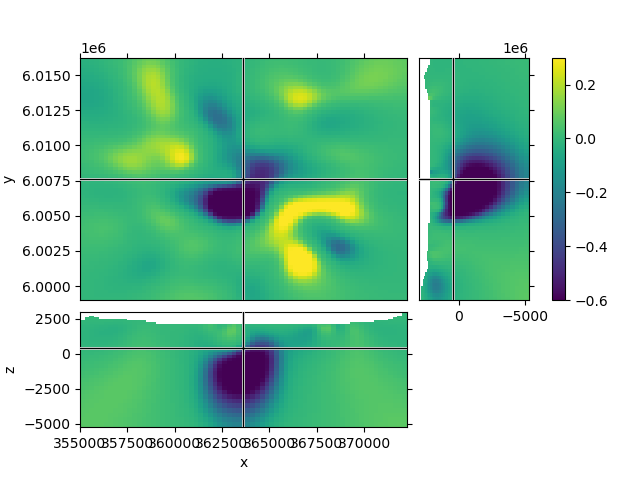### 1.2 Create a function to improve plots, labeling after creation#

Depending on your data the default option might look a bit odd. The look of the figure can be improved by getting its handle and adjust it.

```def beautify(title, fig=None):
"""Beautify the 3D Slicer result."""

# Get figure handle if not provided
if fig is None:
fig = plt.gcf()

# Get principal figure axes
axs = fig.get_children()

# Set figure title
fig.suptitle(title, y=0.95, va="center")

# Adjust the y-labels on the first subplot (XY)
plt.setp(axs.yaxis.get_majorticklabels(), rotation=90)
for label in axs.yaxis.get_ticklabels():
label.set_visible(False)
for label in axs.yaxis.get_ticklabels()[::3]:
label.set_visible(True)
axs.set_ylabel("Northing (m)")

# Adjust x- and y-labels on the second subplot (XZ)
axs.set_xticks([357500, 362500, 367500])
axs.set_xlabel("Easting (m)")

plt.setp(axs.yaxis.get_majorticklabels(), rotation=90)
axs.set_yticks([2500, 0, -2500, -5000])
axs.set_yticklabels(["\$2.5\$", "0.0", "-2.5", "-5.0"])
axs.set_ylabel("Elevation (km)")

# Adjust x-labels on the third subplot (ZY)
axs.set_xticks([2500, 0, -2500, -5000])
axs.set_xticklabels(["", "0.0", "-2.5", "-5.0"])

axs.set_ylabel("Density (g/cc\$^3\$)")

# Ensure sufficient margins so nothing is clipped
```
```mesh.plot_3d_slicer(Lpout)
beautify("mesh.plot_3d_slicer(Lpout)")
```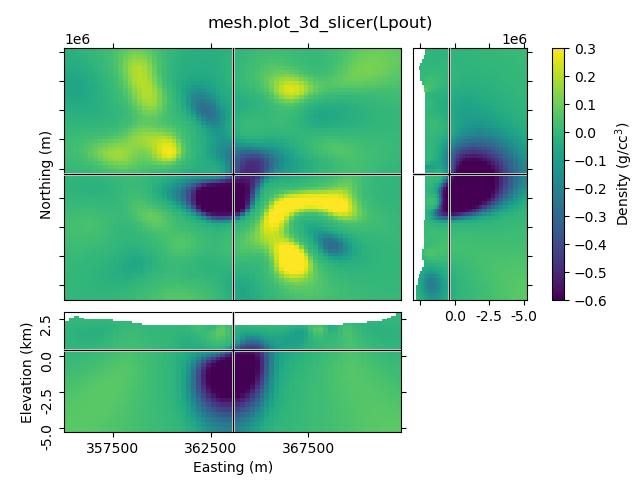### 1.3 Set xslice, yslice, and zslice; transparent region#

The 2nd-4th input arguments are the initial x-, y-, and z-slice location (they default to the middle of the volume). The transparency-parameter can be used to define transparent regions.

```mesh.plot_3d_slicer(Lpout, 370000, 6002500, -2500, transparent=[[-0.02, 0.1]])
beautify(
"mesh.plot_3d_slicer("
"\nLpout, 370000, 6002500, -2500, transparent=[[-0.02, 0.1]])"
)
```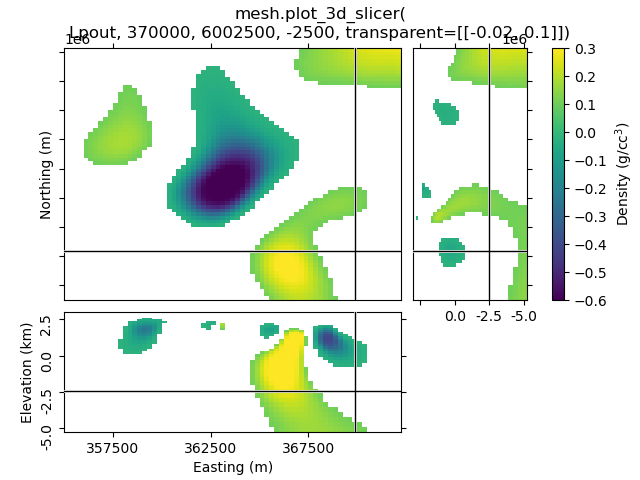### 1.4 Set clim, use pcolor_opts to show grid lines#

```mesh.plot_3d_slicer(
Lpout, clim=[-0.4, 0.2], pcolor_opts={"edgecolor": "k", "linewidth": 0.1}
)
beautify(
"mesh.plot_3d_slicer(\nLpout, clim=[-0.4, 0.2], "
"pcolor_opts={'edgecolor': 'k', 'linewidth': 0.1})"
)
```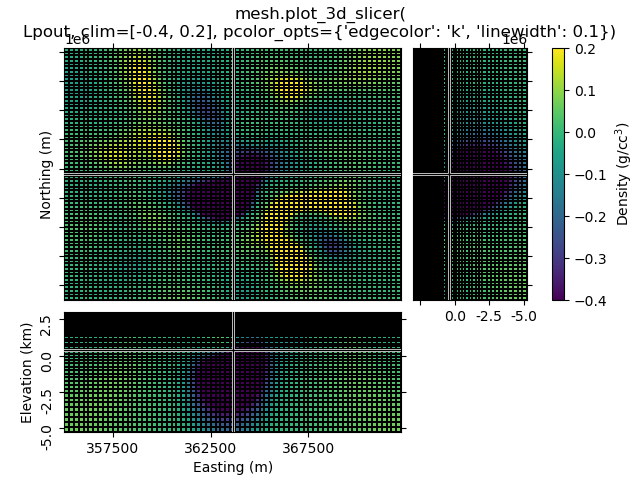### 1.5 Use pcolor_opts to set SymLogNorm, and another cmap#

```mesh.plot_3d_slicer(
Lpout, pcolor_opts={"norm": SymLogNorm(linthresh=0.01), "cmap": "RdBu_r"}
)
beautify(
"mesh.plot_3d_slicer(Lpout,"
"\npcolor_opts={'norm': SymLogNorm(linthresh=0.01),'cmap': 'RdBu_r'})`"
)
```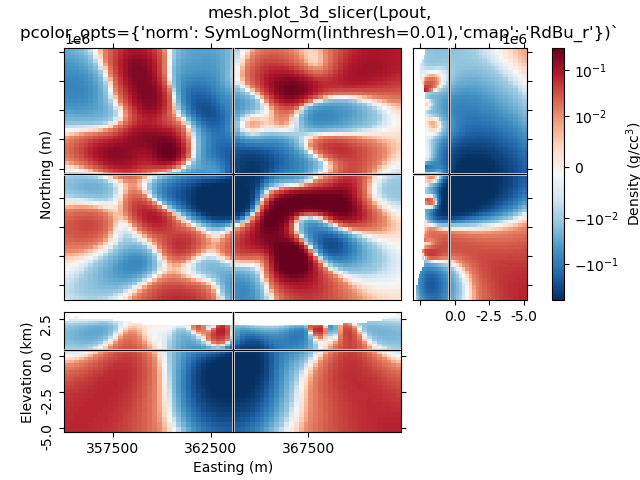### 1.6 Use `aspect` and `grid`#

By default, `aspect='auto'` and `grid=[2, 2, 1]`. This means that the figure is on a 3x3 grid, where the xy-slice occupies 2x2 cells of the subplot-grid, xz-slice 2x1, and the zy-silce 1x2. So the `grid=[x, y, z]`-parameter takes the number of cells for x, y, and z-dimension.

`grid` can be used to improve the probable weired subplot-arrangement if `aspect` is anything else than `auto`. However, if you zoom then it won’t help. Expect the unexpected.

```mesh.plot_3d_slicer(Lpout, aspect=["equal", 1.5], grid=[4, 4, 3])
beautify("mesh.plot_3d_slicer(Lpout, aspect=['equal', 1.5], grid=[4, 4, 3])")
```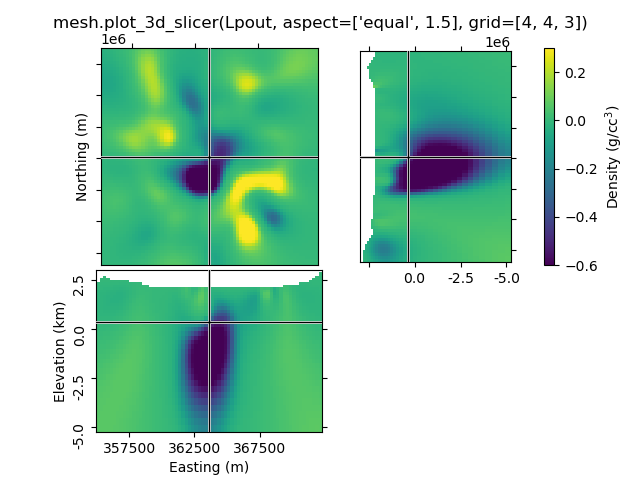### 1.7 Transparency-slider#

Setting the transparent-parameter to ‘slider’ will create interactive sliders to change which range of values of the data is visible.

```mesh.plot_3d_slicer(Lpout, transparent="slider")
beautify("mesh.plot_3d_slicer(Lpout, transparent='slider')")
```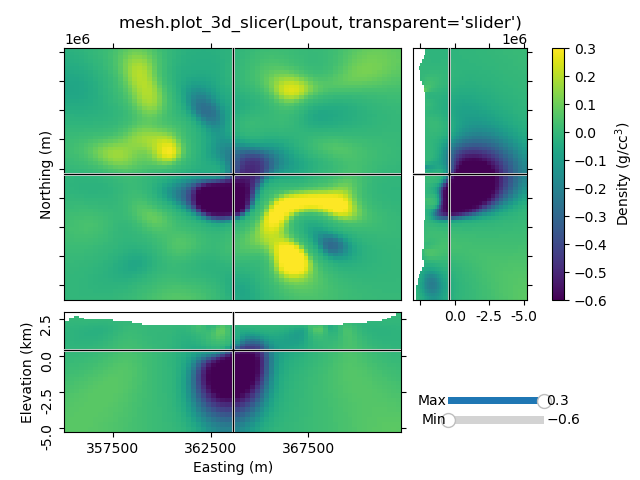## Case 2: Just using the Slicer class#

This way you get the figure-handle, and can do further stuff with the figure.

```# You have to initialize a figure
fig = plt.figure()

# Then you have to get the tracker from the Slicer
tracker = discretize.mixins.Slicer(mesh, Lpout)

# Finally you have to connect the tracker to the figure
fig.canvas.mpl_connect("scroll_event", tracker.onscroll)

# Run it through beautify
beautify("'discretize.mixins.Slicer' together with\n'fig.canvas.mpl_connect'", fig)

plt.show()
```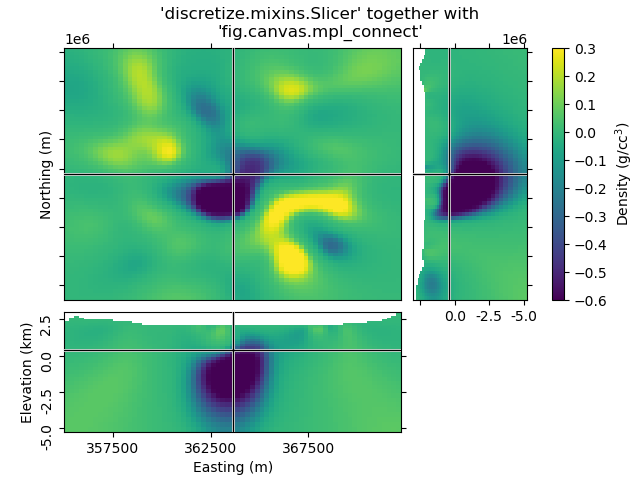Total running time of the script: ( 0 minutes 2.847 seconds)

Gallery generated by Sphinx-Gallery